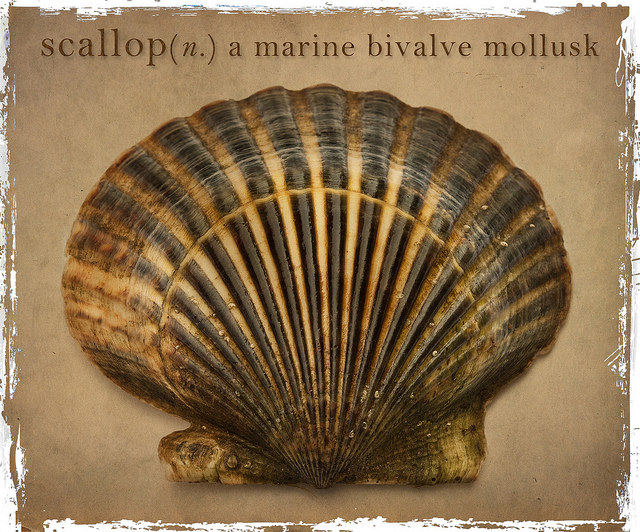# Def Keyword In Python

## Overview:

• def keyword in Python is used for defining a function. It is synonymous with the word definition.• In case of classes the def keyword is used for defining the methods of a class.

• Note that the keyword self needs to be passed as the first parameter in member function definitions.

• def keyword is also required to define special member function of a class like __init__().

## Example 1:

In this example Python program a function is defined using the def keyword.

 import math   def areaOfTheCircle(radius):     return math.pi*radius*radius   radiusValue = 2 circleArea  = areaOfTheCircle(radiusValue) print("Area of the circle with %f units radius is %f units"%(radiusValue, circleArea));

## Output 1:

 Area of the circle with 2.000000 units radius is 12.566371 units

## Example 2:

In this example Python program a method of a class is defined using the def keyword.

 import math   class Circle:     myRadius = 0         def __init__(self, radius):         self.myRadius = radius       def area(self):         return math.pi*self.myRadius*self.myRadius   radius = 5 circle = Circle(radius) print("Area of the circle with %f units radius is %f units"%(radius, circle.area()));

## Output2:

 Area of the circle with 5.000000 units radius is 78.539816 units

Copyright 2019 © pythontic.com